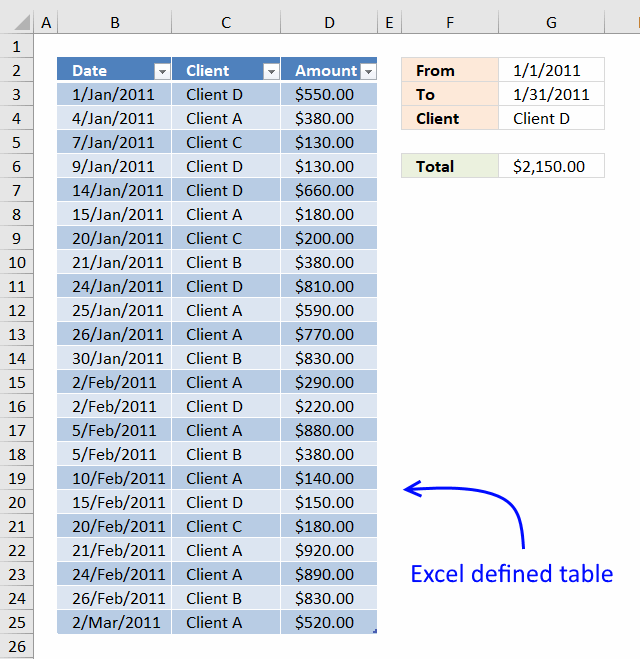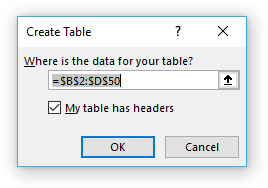Author: Oscar Cronquist Article last updated on January 17, 2019LOVE this example, my issue/need is, I need to add the results. So instead of States and Names, I have to match a client name, and add all the sales totals:

clientA 10
clientA 10
clientA 10
clientB 5
clientB 5
clientB 5

So if I search for clientA, I need one cell that keeps a running total as sales are added. Lastly, a date range will need to be given, so search for sales from clientA between two dates and keep a running total...

I highly recommend a pivot table for this task, however, this article demonstrates a formula combined with an Excel defined table. A pivot table is lightning fast if you have lots of data to work with and is easy to learn.

Formula in cell G6:

=SUMIFS(Table1[Amount], Table1[Client], G4, Table1[Date], "<="&G3, Table1[Date], ">="&G2)

The SUMIFS function was introduced in Excel 2007, use the SUMPRODUCT function if you have an earlier Excel version.

=SUMPRODUCT(Table1[Amount]*(Table1[Client]=G4)*(Table1[Date]<=G3)*(Table1[Date]>=G2))

### How to create an Excel defined table

1. Select a cell in the data set.
2. Press CTRL + T
3. A dialog box appears, enable checkbox if your data set has headers.4. Press with left mouse button on OK.

### Explaining formula in cell G6

Some of the cell references in the SUMIFS function are structured references pointing to a range in an Excel defined table. Structured references adjust automatically when data is added or removed to the table, the formula will instantly return the new running total.

Structured reference -> Table1[Amount]

The SUMIFS function adds numbers based on criteria and returns the total.

SUMIFS(sum_range, criteria_range1criteria1[criteria_range2], [criteria2], ...)

#### Step 1 - First argument is sum_range

The sum_range argument is a structured reference: Table1[Amount]. This cell ranges contains the numbers to be added based on critera.

#### Step 2 - Second and third argument

The first pair of arguments evaluates if cells in column Client equal value in cell G4.

 Argument Cell reference criteria_range1 Table1[Client] criteria1 G4

#### Step 3 - Fourth and fifth argument

The second pair of arguments evaluates if cells in column Date equal or are less than value in cell G3.

 Argument Cell reference criteria_range2 Table1[Date] criteria2 "<="&G3

The less than and equal sign are concatenated to cell reference G3 using the ampersand character.

#### Step 4 - Sixth and seventh argument

The third pair of arguments evaluates if cells in column Date equal or are larger than value in cell G2.

 Argument Cell reference criteria_range3 Table1[Date] criteria3 ">="&G2

The larger than and equal sign are concatenated to cell reference G2 using the ampersand character.

### Get the Excel fileRunning-totals-within-date-range.xlsx